# Grade - math word problems

#### Number of examples found: 5342

• FedorFedor stood in the small pool. Above the surface was a part of his body that was twice as long as part of his body underwater. What height does Fedor have, if his body part below the surface is 5 dm long?
• Inverse matrix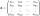Find how many times is the larger determinant is the matrix A, which equals 9 as the determinant of its inverse matrix.
• PercentsHow many percents is 900 greater than the number 750?
• Shell of cylinderCalculate the content of shell the 1.6 m height cylinder with a base radius of 0.4 m.
• Circle r,DCalculate the diameter and radius of the circle if it has length 26.59 cm.
• Area of squareCalculate the content area of the square whose perimeter is 24 dm.
• Cube 6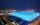Surface area of one wall cube is 1600 cm square. How many liters of water can fit into the cube?
• Diameter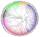The diameter of a circular plot is 14 dm. Find the circumference and area.
• Diamond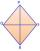Calculate the length of the two diagonals of the diamond if: a = 13 cm v = 12 cm
• Time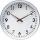16.2 days ..... how many hours is it?
• Potatoes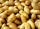Could 446 tons of potatoes (ρ = 771 kg/m³) fits in a warehouse with a volume of 699 m³ ?
• Areaf of ST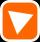It is given square DBLK with side |BL|=13. Calculate area of triangle DKU if vertex U lie on line LB.
• Cyclist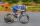A cyclist passes 88 km in 4 hours. How many kilometers he pass in 8 hours?
• ThalesCalculate the length of the Thales' circle described to right triangle with hypotenuse 18.4 cm.
• Chickens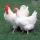2 chickens give 2 eggs in 2 days. How many eggs can give 8 chickens for 8 days?
• Diagonal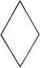Can be a diagonal of diamond twice longer than it side?
• Proof I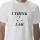When added to the product of two consecutive integers larger one, we get square larger one. Is this true or not?
• Tree trunk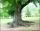Calculate the average tree trunk with a circumference of 149 cm.
• Vector perpendicularFind the vector a = (2, y, z) so that a⊥ b and a ⊥ c where b = (-1, 4, 2) and c = (3, -3, -1)
• Numbers divisionWith what number should be divided mixed number 2 3/4 to get 11/12?

Do you have an interesting mathematical word problem that you can't solve it? Submit a math problem, and we can try to solve it.

We will send a solution to your e-mail address. Solved examples are also published here. Please enter the e-mail correctly and check whether you don't have a full mailbox.

Please do not submit problems from current active competitions such as Mathematical Olympiad, correspondence seminars etc...